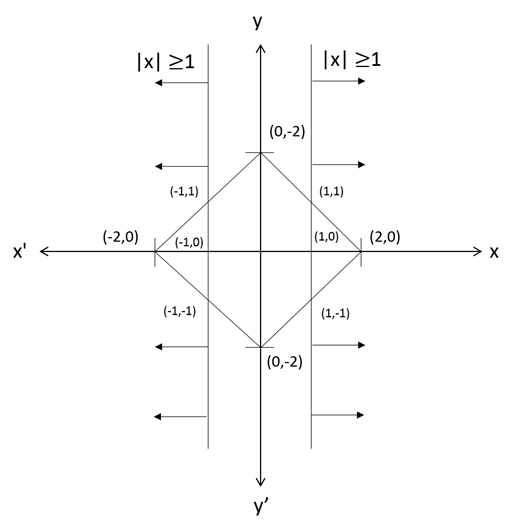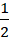# CAT 2019 Question Paper | Quants Slot 1

###### CAT Previous Year Paper | CAT Quants Questions | Question 28

This is an interesting question that appeared in CAT 2019. While it looks tough, on looking further it is a very solvable question. All CAT previous year paper have similar questions that can be solved easily when a small construction is done. Once you arrive at the diagram, it is a very easy question.

Question 28 : Let S be the set of all points (x,y) in the x-y plane such that |x| + |y| ≤ 2 and |x| ≥ 1. Then, the area, in square units, of the region represented by S equals [TITA]

## Best CAT Coaching in Chennai

#### CAT Coaching in Chennai - CAT 2022Limited Seats Available - Register Now!Construct the given data on a rough graph.
Required area = Sum of area of two smaller triangles [ (-2,0) (-1,1) (-1,-1) and (2,0) (1,-1) (1,1) ]
Required area = 2 xx base x height
Required area = 1 x 2 = 2 Sq units

The question is "Let S be the set of all points (x,y) in the x-y plane such that |x| + |y| ≤ 2 and |x| ≥ 1. Then, the area, in square units, of the region represented by S equals [TITA] "

##### Hence, the answer is 2

###### CAT Coaching in ChennaiCAT 2023

Classroom Batches Starting Now! @Gopalapuram

###### Best CAT Coaching in Chennai Introductory offer of 5000/-

Attend a Demo Class

##### Where is 2IIM located?

2IIM Online CAT Coaching
A Fermat Education Initiative,
58/16, Indira Gandhi Street,
Kaveri Rangan Nagar, Saligramam, Chennai 600 093

##### How to reach 2IIM?

Mobile: (91) 99626 48484 / 94459 38484
WhatsApp: WhatsApp Now
Email: info@2iim.com